# CBSE Solutions for Class 10 English

#### GSEB std 10 science solution for Gujarati check Subject Chapters Wise::

The angle of elevation from ground to the top of the building is 30° and the distance between the point and the base of the building is 10m. Find the height of the building.

જવાબ : tanx = Height/Base tan30° = Height/10 Height= 10/√3m

The angle of elevation from ground to the top of the building is 30° and the height of the building is 10/3m. Find the distance between the point and the base of the building.

જવાબ : tanx = Height/Base tan30° =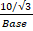Base = 10

The angle of elevation from ground to the top of the building is 60° and the distance between the point and the base of the building is 10m. Find the height of the building.

જવાબ : tanx = Height/Base Tan60° = Height/10 Height= 103m

If the distance between the base of the building and the point is 10m and the height of the building is 10/3m. Find the angle of elevation from ground to the top of the building.

જવાબ : tanx = Height/Base tanx=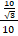= 1/3 x= 30°

The angle of elevation from ground to the top of the building is 60° and the height of the building is 103m. Find the distance between the point and the base of the building.

જવાબ : tanx = Height/Base tan60° =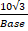Base = 10

If the distance between the base of the building and the point is 10m and the height of the building is 103m. Find the angle of elevation from ground to the top of the building.

જવાબ : tanx = Height/Base tanx=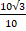= 3 x= 60°

The angle of elevation from ground to the top of the building is 60° and the height of the building is 5√3m. Find the distance between the point and the base of the building.

જવાબ : tanx = Height/Base tan60° =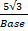Base = 5

The angle of elevation from ground to the top of the building is 30° and the distance between the point and the base of the building is 5m. Find the height of the building.

જવાબ : tanx = Height/Base tan30° = Height/5 Height= 5/3m

The angle of elevation from ground to the top of the building is 60° and the distance between the point and the base of the building is 5m. Find the height of the building.

જવાબ : tanx = Height/Base Tan60° = Height/5 Height= 5√3m

The angle of elevation from ground to the top of the building is 30° and the height of the building is 5/√3m. Find the distance between the point and the base of the building.

જવાબ : tanx = Height/Base tan30° =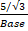Base = 5

If the distance between the base of the building and the point is 5m and the height of the building is 5√3m. Find the angle of elevation from ground to the top of the building.

જવાબ : tanx = Height/Base tanx=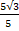= √3 x= 60°

If the distance between the base of the building and the point is 5m and the height of the building is 5/3m. Find the angle of elevation from ground to the top of the building.

જવાબ : tanx = Height/Base tanx=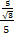= 1/3 x= 30°

The angle of elevation from ground to the top of the building is 30° and the distance between the point and the base of the building is 30m. Find the height of the building.

જવાબ : tanx = Height/Base tan30° = Height/30 Height= 103m

If the distance between the base of the building and the point is 30m and the height of the building is 30/√3m. Find the angle of elevation from ground to the top of the building.

જવાબ : tanx = Height/Base tanx=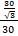= 1/√3 x= 30°

The angle of elevation from ground to the top of the building is 60° and the height of the building is 30√3m. Find the distance between the point and the base of the building.

જવાબ : tanx = Height/Base tan60° =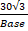Base = 30

The angle of elevation from ground to the top of the building is 30° and the height of the building is 30/3m. Find the distance between the point and the base of the building.

જવાબ : tanx = Height/Base tan30°=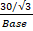Base = 30

The angle of elevation from ground to the top of the building is 60° and the distance between the point and the base of the building is 30m. Find the height of the building.

જવાબ : tanx = Height/Base Tan60° = Height/30 Height=30√3m

The angle of elevation from ground to the top of the building is 30° and the height of the building is 1/√3m. Find the distance between the point and the base of the building.

જવાબ : tanx = Height/Base tan30° =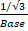Base = 1

The angle of elevation from ground to the top of the building is 30° and the distance between the point and the base of the building is 1m. Find the height of the building.

જવાબ : tanx = Height/Base tan30° = Height/1 Height= 1/√3m

If the distance between the base of the building and the point is 30m and the height of the building is 303m. Find the angle of elevation from ground to the top of the building.

જવાબ : tanx = Height/Base tanx=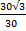= 3 x= 60°

If the distance between the base of the building and the point is 40m and the height of the building is 40m. Find the angle of elevation from ground to the top of the building.

જવાબ : tanx = Height/Base tanx=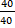= 1 x= 45°

The angle of elevation from ground to the top of the building is 30° and the height of the building is 40/√3m. Find the distance between the point and the base of the building.

જવાબ : tanx = Height/Base tan30° =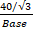• Base = 40

The angle of elevation from ground to the top of the building is 40° and the distance between the point and the base of the building is 10m. Find the height of the building.

જવાબ : tanx = Height/Base tan30° = Height/40 Height= 40/√3m

If the distance between the base of the building and the point is 25m and the height of the building is 25√3m. Find the angle of elevation from ground to the top of the building.

જવાબ : tanx = Height/Base tanx=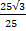= √3 x= 60°

If the distance between the base of the building and the point is 25m and the height of the building is 25/√3m. Find the angle of elevation from ground to the top of the building.

જવાબ : tanx = Height/Base tanx=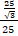= 1/√3 x= 30°

The angle of elevation from ground to the top of the building is 60° and the height of the building is 25√3m. Find the distance between the point and the base of the building.

જવાબ : tanx = Height/Base tan60° =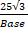Base = 25

The angle of elevation from ground to the top of the building is 60° and the distance between the point and the base of the building is 25m. Find the height of the building.

જવાબ : tanx = Height/Base Tan60° = Height/25 Height= 25√3m

The angle of elevation from ground to the top of the building is 30° and the height of the building is 25/√3m. Find the distance between the point and the base of the building.

જવાબ : tanx = Height/Base tan30° =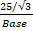Base = 25

The angle of elevation from ground to the top of the building is 30° and the distance between the point and the base of the building is 25m. Find the height of the building.

જવાબ : tanx = Height/Base tan30° = Height/25 Height= 25/√3m

If the distance between the base of the building and the point is 10m and the height of the building is 10m. Find the angle of elevation from ground to the top of the building.

જવાબ : tanx = Height/Base tanx=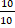= 1 x= 45°

The angle of elevation from ground to the top of the building is 60° and the height of the building is 10m. Find the distance between the point and the base of the building.

જવાબ : tanx = Height/Base Tan45° =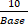Base = 10

The angle of elevation from ground to the top of the building is 45° and the distance between the point and the base of the building is 10m. Find the height of the building.

જવાબ : tanx = Height/Base Tan45° = Height/10 Height= 10m

The angle of elevation from ground to the top of the building is 0° and the height of the building is 10m. Find the distance between the point and the base of the building.

જવાબ : tanx = Height/Base tan0° =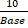Base = 0

The angle of elevation from ground to the top of the building is 0° and the distance between the point and the base of the building is 10m. Find the height of the building.

જવાબ : tanx = Height/Base tan0° = Height/10 Height= 0m

If the distance between the base of the building and the point is 20m and the height of the building is 20√3m. Find the angle of elevation from ground to the top of the building.

જવાબ : tanx = Height/Base tanx=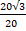= √3 x= 60°

If the distance between the base of the building and the point is 20m and the height of the building is 20/√3m. Find the angle of elevation from ground to the top of the building.

જવાબ : tanx = Height/Base tanx=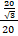= 1/√3 x= 30°

The angle of elevation from ground to the top of the building is 60° and the height of the building is 20√3m. Find the distance between the point and the base of the building.

જવાબ : tanx = Height/Base tan60° =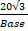Base = 20

The angle of elevation from ground to the top of the building is 60° and the distance between the point and the base of the building is 20m. Find the height of the building.

જવાબ : tanx = Height/Base Tan60° = Height/20 Height= 20√3m

The angle of elevation from ground to the top of the building is 30° and the height of the building is 20/√3m. Find the distance between the point and the base of the building.

જવાબ : tanx = Height/Base tan30° =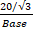Base = 20

The angle of elevation from ground to the top of the building is 30° and the distance between the point and the base of the building is 20m. Find the height of the building.

જવાબ : tanx = Height/Base tan30° = Height/20 Height= 20/√3m

If the distance between the base of the building and the point is 100m and the height of the building is 100√3m. Find the angle of elevation from ground to the top of the building.

જવાબ : tanx = Height/Base tanx=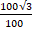= √3 x= 60°

If the distance between the base of the building and the point is 100m and the height of the building is 100/√3m. Find the angle of elevation from ground to the top of the building.

જવાબ : tanx = Height/Base tanx=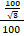= 1/√3 x= 30°

The angle of elevation from ground to the top of the building is 60° and the height of the building is 100√3m. Find the distance between the point and the base of the building.

જવાબ : tanx = Height/Base tan60° =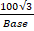Base = 100

The angle of elevation from ground to the top of the building is 60° and the distance between the point and the base of the building is 100m. Find the height of the building.

જવાબ : tanx = Height/Base Tan60° = Height/100 Height= 100√3m

The angle of elevation from ground to the top of the building is 30° and the height of the building is 100/√3m. Find the distance between the point and the base of the building.

જવાબ : tanx = Height/Base tan30° =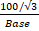Base = 100

The angle of elevation from ground to the top of the building is 30° and the distance between the point and the base of the building is 100m. Find the height of the building.

જવાબ : tanx = Height/Base tan30° = Height/100 Height= 100/√3m

If the distance between the base of the building and the point is 1m and the height of the building is 1√3m. Find the angle of elevation from ground to the top of the building.

જવાબ : tanx = Height/Base tanx=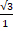= √3 x= 60°

If the distance between the base of the building and the point is 1m and the height of the building is 1/√3m. Find the angle of elevation from ground to the top of the building.

જવાબ : tanx = Height/Base tanx=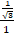= 1/√3 x= 30°

The angle of elevation from ground to the top of the building is 60° and the height of the building is 1√3m. Find the distance between the point and the base of the building.

જવાબ : tanx = Height/Base tan60° =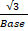Base = 1

The angle of elevation from ground to the top of the building is 60° and the distance between the point and the base of the building is 1m. Find the height of the building.

જવાબ : tanx = Height/Base Tan60° = Height/1 Height= 3m

A ladder 15 m long just reaches the top of a vertical wall. If the ladder makes an angle of 60° with the wall, then calculate the height of the wall. (2013OD)

જવાબ :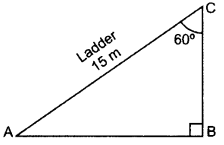BAC = 180° – 90° – 60° = 30°
sin 30° = BC/AC
1/2=BC/15
2BC = 15
BC = 15/2m

In the given figure, a tower AB is 20 m high and BC, its shadow on the ground, is 20√3m long. Find the Sun’s altitude. (2015OD)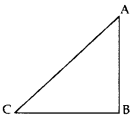જવાબ : AB = 20 m, BC =20√3m,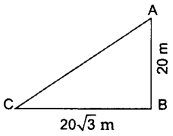In ∆ABC, Using trigonometry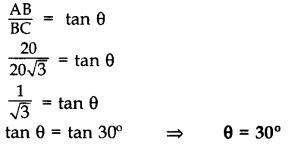​​​​​​​

In the figure, AB is a 6 m high pole and CD is a ladder inclined at an angle of 60° to the horizontal and reaches up to a point D of pole. If AD = 2.54 m. Find the length of the ladder. (Use √3 = 1.73) (2016D)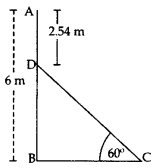જવાબ : BD = AB – AD = 6 – 2.54 = 3.46 m
In rt., ∆DBC, sin 60° = BD/DC
√3/2=3.46/DC
√3 /DC = 3.46 x 2
Length of the ladder, DC = 6.92 /√3=6.92/1.73
DC = 4 m

A ladder, leaning against a wall, makes anangle of 60° with the horizontal. If the foot of the ladder is 2.5 m away from the wall, find the length of the ladder. (2016OD)

જવાબ : Let us assume AC be the ladder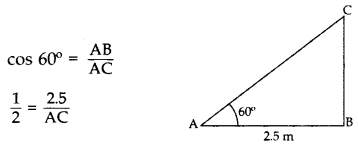Length of ladder, AC = 5 m 2.5 m

If a tower 30 m high, casts a shadow 10√3m long on the ground, then what is the angle of elevation of the sun? (2017OD)

જવાબ : Let us assume required angle be θ.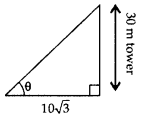tan θ = 30 /10 √3
tan θ = √3
tan θ = tan 60°

θ = 60°

The angle of elevation of an aeroplane from a point on the ground is 60°. After a flight of 30 seconds the angle of elevation becomes 30°. If the aeroplane is flying at a constant height of 3000√ 3 m, find the speed of the aeroplane. (2014OD)

જવાબ : Let us assume that A be the point on the ground and C be the aeroplane.
In ∆ABC,From the top of a 60 m high building, the angles of depression of the top and the bottom of a tower are 45° and 60° respectively. Find the height of the tower. (Take √3 = 1.73] (2014OD)

જવાબ : Let us assume that AC be the building & DE be the tower.Height of the tower, DE = BC = DE = AC – AB
DE = 60 – 20$\sqrt{3}$ = 20(3 – $\sqrt{3}$)
DE = 20(3 – 1.73) = 20(1.27)
DE =
25.4 m

The angle of elevation of the top of a building from the foot of the tower is 30° and the angle of elevation of the top of the tower from the foot of the building is 45°. If the tower is 30 m high, find the height of the building. (2015D)

જવાબ :The angle of elevation of an aeroplane from a point A on the ground is 60°. After a flight of 15 seconds, the angle of elevation changes to 30°. If the aeroplane is flying at a constant height of 1500√3 m, find the speed of the plane in km/hr. (2015OD)

જવાબ : Let us assume that AL = x, BL = CM = 1500√3The angles of depression of the top and bottom of a 50 m high building from the top of a tower are 45° and 60° respectively. Find the height of the tower and the horizontal distance between the tower and the building. [Use √3 = 1.73]. (2016D)

જવાબ :Let us assume that AE be the building and CD be the tower.

height of the tower = h m and, the horizontal distance between tower and building = x m …[Given in the ques.]
BD = AE = 50 m

=> BC = CD – BD = (h – 50) mFrom (i),

x = h – 50 = 118.25 – 50 = 68.25 m
Height of the tower, h = 118.25 m
Horizontal distance between tower and Building, x = 68.25 m

A man standing on the deck of a ship, which is 10 m above water level, observes the angle of elevation of the top of a hill as 60° and the angle of depression of the base of hill as 30°. Find the distance of the hill from the ship and the height of the hill. (2016D)

જવાબ :Let the man standing on the deck of a ship be at point A and let CE be the hill.
Here BC is the distance of hill from ship and CE be the height of hill.
In rt.
ABC, tan 30° = AB/BC
BC = 10√3 m .(A)
BC = 10(1.73) = 17.3 m
AD = BC = 10√3m …(B) [From (A)
In rt. ∆ADE, tan 60° = DE/AD

√3 =DE/10 √3 … [From (B)
DE = 10√3 × √3 = 30 m
CE = CD + DE = 10 + 30 = 40 m
Hence, the distance of the hill from the ship is 17.3 m and the height of the hill is 40 m
.

The tops of two towers of height x and y, standing on level ground, subtend angles of 30° and 60° respectively at the centre of the line joining their feet, then find x : y. (2015D)

જવાબ : Let the base of towers be k.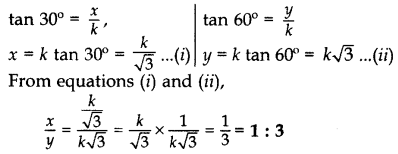The angles of depression of two ships from the top of a light house and on the same side of it are found to be 45° and 30°. If the ships are 200 m apart, find the height of the light house. (2012D)

જવાબ : Let us assume that  AB be the height of the light house,
D and C are two ships

• DC = 200 m
Let BC = x m, AB = h m
In rt. ∆ABC,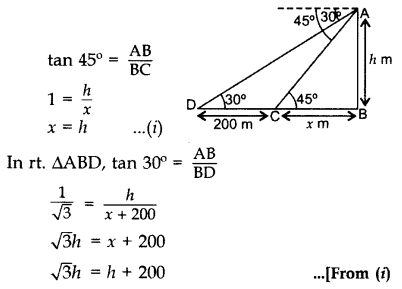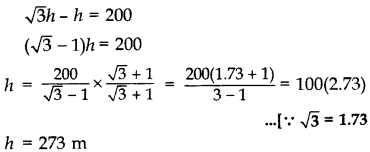Height of the light house = 273 m

The horizontal distance between two poles is 15 m. The angle of depression of the top of first pole as seen from the top of second pole is 30°. If the height of the second pole is 24 m, find the height of the first pole. [Use $\sqrt{3}$ = 1.732] (2013D)

જવાબ : Let us assume that AB be the 1st pole
and CE be the 2nd pole = 24 m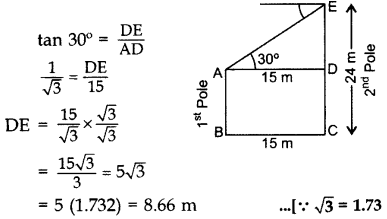=> Height of the first pole,
AB = CD = CE – DE
= 24 – 8.66 = 15.34 m

As observed from the top of a 60 m high light house from the sea-level, the angles of depression of two ships are 30° and 45°. If one ship is exactly behind the other on the same side of the light-house, find the distance between the two ships. (Use √3 = 1.732] (2013OD)

જવાબ : Let AB = 60 m be the height of Light-house and C and D be the two ships.
In right ∆ABC,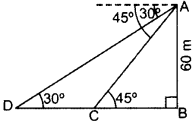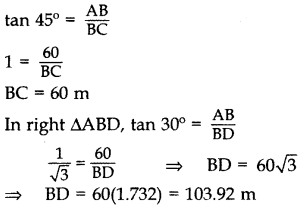=>  Distance between the two ships = CD = BD – BC = 103.92 – 60 = 43.92 m

Two ships are there in the sea on either side of a light house in such a way that the ships and the light house are in the same straight line. The angles of depression of two ships as observed from the top of the light house are 60° and 45°. If the height of the light house is 200 m, find the distance between the two ships. [Use √3 = 1.73] (2014D)

જવાબ :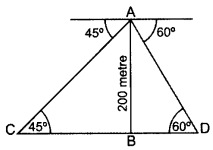Let us assume thzat AB be the light house and C and D be the two ships,
In ∆ABC, tan 45° = AB/BC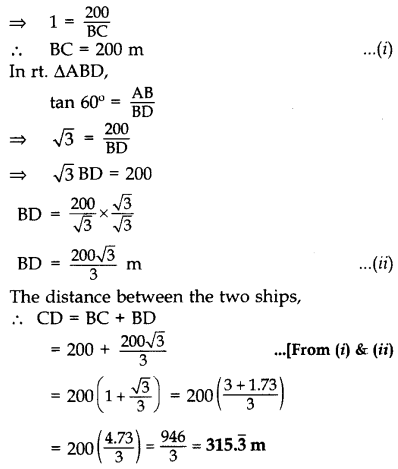A kite is flying at a height of 75m from the level ground, attached to a string inclined at 60° to the horizontal. Find the length of the string assuming that there is no slack in it.જવાબ : Letus assume OB be the length of the string from the level of ground and O be the point of the observer, then, AB = 75m and  OAB = 90° and AOB = 60°, let OB = l meters.

From the right triangle OAB, we haveAn observer 1.5 m tall is 30 m away from a chimney. The angle of elevation of the top of the chimney from his eye is 60°. Find the height of the chimney.

જવાબ :The angles of elevation of the top of a tower from two points at distances of 5 metres and 20 metres from the base of the tower and in the same straight line with it, are complementary. Find the height of the tower.

જવાબ :The angle of elevation of the top of a tower at a distance of 120 m from a point A on the ground is 45°. If the angle of elevation of the top of a flagstaff fixed at the top of the tower, at A is 60°, then find the height of the flagstaff.જવાબ :### There are No Content Availble For this ChapterA=30° and AB=3cm

 1    ∠C A    2√3  cm 2    AC B    √3 cm 3    BC C    60° 4    ∠B D    90°

જવાબ :

1-C, 2-A, 3-B, 4-DAB/BC= 1/√3 and AB = √3cm

 1 BC A 60° 2 AC B 3 cm 3 ∠A C 30° 4 ∠C D 2√3cm

જવાબ :

1-B, 2-D, 3-A, 4-CC=60° and BC= 3 cm

 1 ∠A A 2√3  cm 2 AB B 30° 3 AC C 90° 4 ∠B D 3cm

જવાબ :

1-B, 2-D, 3-A, 4-CC=45° and BC= 3 cm

 1 ∠A A 90° 2 AB B 45° 3 AC C 3 cm 4 ∠B D √6

જવાબ :

1-B, 2-C, 3-D, 4-AB= 90° and AB=BC, BC= 10 cm

 1 ∠A A 45° 2 AC B 10 cm 3 AB C 2√5

જવાબ :

1-A, 2-C, 3-BC=30° and AC=6cm

 1 ∠A A 3√3 cm 2 ∠B B 3 cm 3 AB C 90° 4 BC D 60°

જવાબ :

1-D, 2-C, 3-B, 4-AA=60° and AB=3cm

 1 ∠C A 6cm 2 ∠B B 3√3cm 3 AC C 90° 4 BC D 30°

જવાબ :

1-D, 2-C, 3-A, 4-BC=30° and AB=3cm

 1 ∠A A 6cm 2 ∠B B 3√3cm 3 AC C 90° 4 BC D 60°

જવાબ :

1-D, 2-C, 3-A, 4-BAB/BC= 1 and AC= 4√2cm

 1 AB A 90° 2 ∠A B 4 cm 3 AB/AC C 45° 4 ∠B D 1/√2

જવાબ :

1-B, 2-C, 3-D, 4-AAB/BC= √3 and AB = √3cm

 1 BC A 2cm 2 AC B 30° 3 ∠A C 1 cm 4 ∠C D 60°

જવાબ :

1-C, 2-A, 3-B, 4-D

### Take a Test

Choose your Test :

### Some Applications of Trigonometry

Math
Chapter 09 : Some Applications of  Trigonometry

### Browse & Download CBSE Books For Class 10 All Subjects

The GSEB Books for class 10 are designed as per the syllabus followed Gujarat Secondary and Higher Secondary Education Board provides key detailed, and a through solutions to all the questions relating to the GSEB textbooks.

The purpose is to provide help to the students with their homework, preparing for the examinations and personal learning. These books are very helpful for the preparation of examination.

For more details about the GSEB books for Class 10, you can access the PDF which is as in the above given links for the same.